# Thue-Morse Navigating Turtles

Here’s a simple rule to generate a pretty sequence of 1s and 0s: Start with the list 1 (just one digit) and repeatedly form a new list by flipping all the bits (1s become 0s and vice versa) and attaching this to the end: $$1 \to 10 \to 1001 \to 10010110 \to 1001011001101001 \to \cdots.$$ For example, to get from 1001 to the next sequence, we swap 1s and 0s to form 0110, and then we join these together to make 10010110. By continuing this process we can make our list longer and longer, and the limiting sequence $$1001011001101001011010011001011001101\cdots$$ is called the Thue-Morse Sequence.

What did I mean by calling this sequence “pretty”? Well, what does this sequence “look like”? One way to visualize it is with a turtle program. Imagine a turtle (let’s call him Leo) walking along the plane, and treat the digits of the Thue-Morse sequence as a list of instructions for the turtle as follows: When Leo sees the digit 1, he steps forward one foot; a 0 tells Leo to turn left by 60 degrees. What does Leo’s path look like? Here are the first 32 instructions he follows: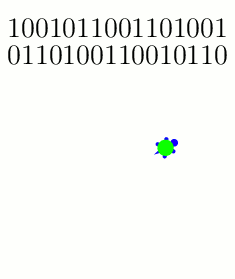The turtle follows these instructions on the first 32 entries of the Thue-Morse sequence: {1: Step; 0: Turn 60}.

And here are snapshots of his path as he gets farther into the Thue-Morse sequence:

Why did we pick these instructions? What if Leo turns 120 degrees instead of 60 degrees when he sees a 0?The turtle follows the Thue-Morse sequence with a slightly different set of instructions: {1: Step; 0: Turn 120}. Shown here after 2^6, 2^8, 2^10, and 2^12 instructions, respectively.

If you zoom out and/or squint a little, these paths are roughly following a famous fractal called the Koch curve. And indeed, the more steps you draw, the closer the resemblance will become (as analyzed in these two papers: [MH2005] and [KZH2008]). But why stop here? What happens if we change the turtle’s instructions even more? I played around with some new rule sets, and these too show how the Koch curve seems innately “programmed” into the structure of the Thue-Morse sequence:Three more pretty examples of the Thue-Morse sequence's "turtle power." In all three cases, the trajectories converge to the Koch curve. Left: {1: (Turn 180, Step); 0: (Turn 60, Step)}, after 2^8 instructions. Middle: {1: (Turn 180, Step); 0: (Turn 120, Step)}, after 2^10 instructions. Right: {1: Turn 180; 0: (Turn 15, Step)}, after 2^10 instructions.

Here’s a final challenge: if the Koch curve and the Thue-Morse sequence are so intimately related, shouldn’t we be able to find some turtle program instructions that trace the Koch curve without “squinting”? Specifically, the Koch curve is usually constructed as the limit of the following sequence of paths, where each iteration is made by stitching together four copies of the previous curve:The Koch curve is constructed as the limit of these paths, where each curve is formed from four (shrunken) copies of the previous curve.

Is there a set of turtle instructions whose path traces exactly these curves? In fact, there is! These instructions work: {1: (Step, Turn 60); 0: Turn 180}.

# Spherical Surfaces and Hat Boxes

To round off our series on round objects (see the first and second posts), let’s compute the sphere’s surface area. We can compute this in the same way we related the area and circumference of a circle two weeks ago. Approximate the surface of the sphere with lots of small triangles, and connect these to the center of the sphere to create lots of triangular pyramids. Each pyramid has volume $$\frac{1}{3}(\text{area of base})(\text{height})$$, where the heights are all nearly $$r$$ and the base areas add to approximately the surface area. By using more and smaller triangles these approximations get better and better, so the volume of the sphere is $$\frac{4}{3}\pi r^3 = \frac{1}{3}(\text{surface area})\cdot r,$$ meaning the surface area is $$4\pi r^2$$. (This and previous arguments can be made precise with the modern language of integral calculus.)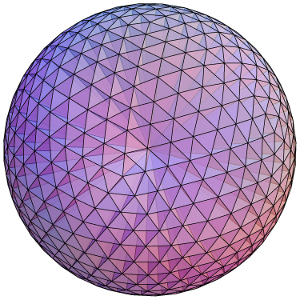Dividing a sphere into many pyramids connected to the center allows us to relate the sphere's surface area and volume.

Here’s an elegant way to rephrase this result: The surface area of a sphere is equal to the area of the curved portion of a cylinder that exactly encloses the sphere. In fact, something very surprising happens here!:

Archimedes’ Hat-Box Theorem: If we draw any two horizontal planes as shown below, then the portions of the sphere and the cylinder between the two planes have the same surface area.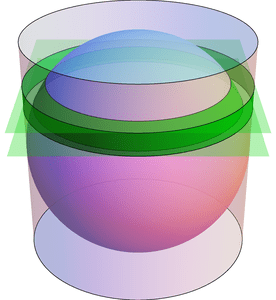Any two horizontal planes cut off a band on the sphere and another band on the enclosing cylinder. Archimedes' Hat-Box Theorem says that these bands have the same area. (The planes shown here have heights $$0.4\cdot r$$ and $$0.6\cdot r$$ above the equator.)

We can prove this with (all!) the methods in the last few posts; here’s a quick sketch. To compute the area of the “spherical band” (usually called a spherical zone), first consider the solid spherical sector formed by joining the spherical zone to the center: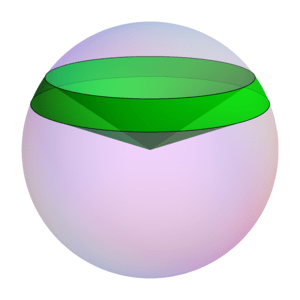The Hat-Box theorem can be proved by relating the area of the spherical zone to the volume of this spherical sector.

By dividing this into lots of triangular pyramids as we did with the sphere above, we can compute the area of the spherical zone by instead computing the sector’s volume. This volume can be computed by breaking it into three parts: two cones and the spherical segment between the two planes (on the left of the next figure). Compute the volume of the spherical segment by comparing (via Cavalieri’s Principle) to the corresponding part of the vase (from the previous post), which can be expressed with just cylinders and cones.

See if you can fill in the details!

# Slicing Spheres

Last week we saw how to compute the area of a circle from first principles. What about spheres?

To compute the volume of a sphere, let’s show that a hemisphere (with radius $$r$$) has the same volume as the vase shown in the figure below, formed by carving a cone from the circular cylinder with radius and height $$r$$. Why this shape? Here’s why: if we cut these two solids at any height $$h$$ (between 0 and $$r$$), the areas of the two slices match. Indeed, the slice—usually called cross section—of the sphere is a circle of radius $$\sqrt{r^2-h^2}$$, which has area $$\pi(r^2-h^2)$$. Similarly, the vase’s cross section is a radius $$r$$ circle with a radius $$h$$ circle cut out, so its area is $$\pi r^2-\pi h^2$$, as claimed.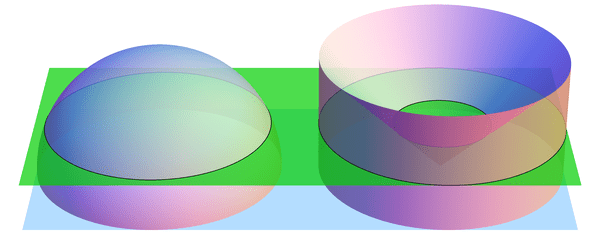When sliced by a horizontal plane at any height $$h$$, the hemisphere and vase have equal cross-sectional areas. (Shown here for $$h = 0.4\cdot r$$.) By Cavalieri's Principle, this implies that they have equal volumes.

If we imagine the hemisphere and vase as being made from lots of tiny grains of sand, then we just showed, intuitively, that the two solids have the same number of grains of sand in every layer. So there should be the same number of grains in total, i.e., the volumes should match. This intuition is exactly right:

Cavalieri’s Principle: any two shapes that have matching horizontal cross sectional areas also have the same volume.

So the volumes are indeed equal, and all that’s left is to compute the volume of the vase. But we can do this! Recall that the cone has volume $$\frac{1}{3} (\text{area of base}) (\text{height}) = \frac{1}{3}\pi r^3$$ (better yet, prove this too! Hint: use Cavalieri’s Principle again to compare to a triangular pyramid). Likewise, the cylinder has volume $$(\text{area of base}) (\text{height}) = \pi r^3$$, so the vase (and hemisphere) have volume $$\pi r^3 – \frac{1}{3} \pi r^3 = \frac{2}{3}\pi r^3$$. The volume of the whole sphere is thus $$\frac{4}{3}\pi r^3$$. Success!

The following visualization illustrates what we have shown, namely $$\text{hemisphere} + \text{cone} = \text{cylinder}.$$ The “grains of sand” in the hemisphere are being displaced horizontally by the stabbing cone, and at the end we have exactly filled the cylinder.

# Archimedes’ Circular Reasoning

Every geometry textbook has formulas for the circumference ($$C = 2 \pi r$$) and area ($$A = \pi r^2$$) of a circle. But where do these come from? How can we prove them?

Well, the first is more a definition than a theorem: the number $$\pi$$ is usually defined as the ratio of a circle’s circumference to its diameter: $$\pi = C/(2r)$$. Armed with this, we can compute the area of a circle. Archimedes’ idea (in 260 BCE) was to approximate this area by looking at regular $$n$$-sided polygons drawn inside and outside the circle, as in the diagram below. Increasing $$n$$ gives better and better approximations to the area.The n-sided regular polygons inside (blue) and outside (red) a circle can be used to approximate the area of the circle. Shown here for n=12.

Look first at the inner polygon. Its perimeter is slightly less than the circle’s circumference, $$C = 2 \pi r$$, and the height of each triangle is slightly less than $$r$$. So when reassembled as shown, the triangles form a rectangle whose area is just under $$C/2\cdot r = \pi r^2$$. Likewise, the outer polygon has area just larger than $$\pi r^2$$. As $$n$$ gets larger, these two bounds get closer and closer to $$\pi r^2$$, which is therefore the circle’s area.

Archimedes used this same idea to approximate the number $$\pi$$. Not only was he working by hand, but the notion of “square root” was not yet understood well enough to compute with. Nevertheless, he was amazingly able to use 96-sided polygons to approximate the circle! His computation included impressive dexterity with fractions: for example, instead of being able to use $$\sqrt{3}$$ directly, he had to use the (very close!) approximation $$\sqrt{3} > 265/153$$. In the end, he obtained the bounds $$3\frac{10}{71} < \pi < 3\frac{1}{7}$$, which are accurate to within 0.0013, or about .04%. (In fact, he proved the slightly stronger but uglier bounds $$3\frac{1137}{8069} < \pi < 3\frac{1335}{9347}$$. See this translation and exposition for more information on Archimedes’ methods.)

These ideas can be pushed further. Focus on a circle with radius 1. The area of the regular $$n$$-sided polygon inscribed in this circle can be used as an approximation for the circle’s area, namely $$\pi$$. This polygon has area $$A_n = n/2 \cdot \sin(360/n)$$ (prove this!). What happens when we double the number of sides? The approximation changes by a factor of $$\frac{A_{2n}}{A_n} = \frac{2\sin(180/n)}{\sin(360/n)} = \frac{1}{\cos(180/n)}.$$ Starting from $$A_4 = 2$$, we can use the above formula to compute $$A_8,A_{16},A_{32},\ldots$$, and in the limit we find that $$\pi = \frac{2}{\cos(180/4)\cdot\cos(180/8)\cdot\cos(180/16)\cdots}.$$ Finally, recalling that $$\cos(180/4) = \cos(45) = \sqrt{\frac{1}{2}}$$ and $$\cos(\theta/2) = \sqrt{\frac{1}{2}(1+\cos\theta)}$$ (whenever $$\cos(\theta/2) \ge 0$$), we can rearrange this into the fun infinite product $$\frac{2}{\pi} = \sqrt{\frac{1}{2}} \cdot \sqrt{\frac{1}{2}+\frac{1}{2}\sqrt{\frac{1}{2}}} \cdot \sqrt{\frac{1}{2}+\frac{1}{2}\sqrt{\frac{1}{2}+\frac{1}{2}\sqrt{\frac{1}{2}}}} \cdots$$ (which I found at Mathworld). (It’s ironic that this formula for a circle uses so many square roots!)

# Voronoi Cells on a Plane

As a pilot of an (oversimplified) airplane, you may want to know how to communicate with the nearest control tower to receive information on local weather, air traffic, and so on. Each control tower has some region of the map that it “controls,” i.e., where it is closer than any other tower. An example is drawn below. These regions are known as the Voronoi regions (or Voronoi cells) of the points, and the whole diagram is the Voronoi decomposition determined by those points.

For a given set of points, we can imagine the corresponding Voronoi regions as follows: grow disks around the points at the same rate, stopping when they hit each other. A disk collides with other disks exactly when its center ceases to be closest, so these regions are indeed the Voronoi cells.

Now let’s kick this into third gear—by which I mean the third dimension. In the animation above, suppose that the circles were moving downward over time, producing a cone below each point. By looking at these cones from above, we see exactly the Voronoi regions! Since cones are easy for computers to render, this provides a handy trick for quickly drawing Voronoi decompositions. In fact, this is how I drew all of these images!By placing identical, vertical cones under the points, one sees the corresponding Voronoi regions from directly above.

This construction is not only of theoretical value, but has also been put to good use packaging Ferrero Rocher chocolates:

Who knew these (delicious!) spherical candies were so mathematical?

# Kissing Spheres

If you put a quarter flat on a table, you can surround it with six other quarters that all touch the original. Is this the best you can do, if the coins can’t overlap? Well, yes: the coins are packed as tightly as possible, so there is no room for a seventh coin to fit around the middle one. We call this the Kissing Number Problem, and it gets much more interesting if you jump from two dimensions to three: given a sphere of radius 1, how many nonoverlapping spheres (of radius 1) can you place around it such that they all “kiss” the original sphere? Said differently, what is the kissing number in 3 dimensions?

Here’s a pretty good attempt: If you put one sphere at each vertex of a cuboctahedron like this,Twelve spheres all kissing a central (blue) sphere and arranged at the vertices of a cuboctahedron.

you’ll end up with 12 that seem pretty tightly packed. In another try, you could put a sphere at each vertex of an icosahedron: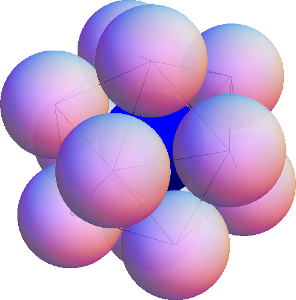Twelve spheres all kissing a central (blue) sphere and arranged at the vertices of an icosahedron.

You’ll again get 12, but this time there is noticable wiggle room to move the spheres without losing contact with the central sphere—so much wiggle room, in fact, that any two spheres can switch places! Is there enough extra space for a 13th sphere to fit? This was a topic of heated debate between Isaac Newton and David Gregory (who believed 12 and 13, respectively), but it was not resolved until much later, in 1953, in favor of Newton’s 12. The proof is highly nontrivial.

What about still higher dimensions? What is the kissing number in four dimensions? (What does a 4-dimensional sphere even look like? Perhaps a topic of a future post…) This too is known, and the answer is 24—the proof is again quite difficult. See if you can figure out the arrangement! What about dimension 5, 6, 7, … ? As might be expected, the problem gets even harder in higher dimensions, and no other exact kissing numbers are known—except for two. Due to some veritable mathematical miracles (namely, the highly symmetric structures called the E8 lattice and the Leech lattice), the kissing numbers in dimensions 8 and 24 have been shown to be 240 and 196560, respectively.

# Teach an Old Dog New Trigs

If you studied your Trig tables in high school, you probably remember some facts like \begin{align*} \cos(30) &= \sqrt{3}/2,\\ \cos(45) &= \sqrt{2}/2, \text{ and}\\ \cos(60) &= 1/2. \end{align*} With a few formulas you can get at a few more angles, e.g., $$\cos(15) = \cos(45 – 30) = \cos(45) \cos(30) + \sin(45) \sin(30) = \frac{ \sqrt{6} + \sqrt{2} }{4},$$ and likewise $$\cos(75) = (\sqrt{6} – \sqrt{2})/4$$.

I now offer two more angles that are not as straightforward but nevertheless have surprisingly nice trig values: 18 and 36. Specifically, we have $$\cos(36) = \frac{1 + \sqrt{5}}{4} = \frac{\phi}{2}, \text{ and } \sin(18) = \frac{-1 + \sqrt{5}}{4} = \frac{1}{2 \phi},$$ where $$\phi = (1 + \sqrt{5})/2$$ is the golden ratio. Try to prove these! (Here’s a hint: Draw a 36-72-72 triangle, and bisect one of the large angles to form a 36-36-108 triangle and a smaller 36-72-72 triangle. Both are isosceles! And one of them is similar to the original triangle!)

You may ask for which other angles we can get “nice” answers. Well, this at least lets us get 18 – 15 = 3 degrees, and therefore any multiple of 3 degrees, as long as we don’t mind multiply nested square roots. But if we’re allowing this then there are even more! For example, Gauss showed that \begin{align*}\cos(360/17) &= \frac{1}{16}\bigg( -1 + \sqrt{17} + \sqrt{34-2 \sqrt{17}} \\ & \qquad {} + 2 \sqrt{ 17 + 3 \sqrt{17} – \sqrt{34-2 \sqrt{17}} – 2 \sqrt{34+2 \sqrt{17}} } \bigg),\end{align*} and there are similar (lengthier!) formulas for $$\cos(360/257)$$ and $$\cos(360/65537)$$ using just nested square roots. Why these denominators? You’ll have to ask our friends Fermat and Galois.